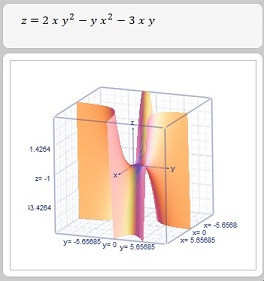# Find all four critical points of f(x,y) = 2xy^2 x^2y 3xy .

## Question:

Find all four critical points of {eq}f(x,y) = 2xy^2 - x^2y-3xy {/eq}.

## Critical Points of a Surface:

A continuous differentiable three dimensional surface represented by an equation of the form, {eq}z = f(x,y) {/eq} attains its optimum values at its interior critical points where its first order partial derivatives all vanish or not all of them exist, or at boundary points. The function may attain a minimum or maximum values at these critical points. We normally evaluate the function at these points, and at all its boundary points, then select the greatest and smallest as the absolute maximum and absolute minimum for the function.

We are required to find the critical points of the function {eq}f(x,y) = 2xy^2 - x^2y-3xy {/eq}.

A critical point is where either both the first order partial derivatives vanish or not both exist. We find the first order partial derivatives of the given function as follows:

{eq}\begin{align*} z &= f(x,y) = 2xy^2 - x^2y-3xy \\ z_x &= 2y^2 -2xy - 3y \\ z_y &= 4xy -x^2 -3x \end{align*} {/eq}

We set the above first order partial derivatives to zero and solve for {eq}x, y {/eq} to find the critical points of the given function.

{eq}\begin{align*} z_x &= 2y^2 -2xy - 3y = 0 \\ 0 &= y( 2y - 2x - 3) \\ y &= 0, \; 2y - 2x - 3 = 0 \\ z_y &= 4xy -x^2 -3x \\ 0 &= x( 4y - x - 3) \\ x &= 0, \; 4y - x - 3 = 0 \end{align*} {/eq}

We have two possible critical points where one of the two or both is true , {eq}x = 0, \; y = 0 {/eq}

We must solve the following two simultaneous equations,

{eq}\begin{align*} 2y - 2x - 3 = 0 \\ 4y - x - 3 = 0 \end{align*} {/eq}

Multiplying the second equation by 2 and subtracting from the first equation we get,

{eq}\begin{align*} 2y - 2x - 3 &= 0 \\ -8y + 2x + 6 &= 0 \\ -6y + 3 &= 0 \\ y &= \frac{1}{2} \\ \end{align*} {/eq}

Substituting the above value for {eq}y {/eq} in the first equation we get,

{eq}\begin{align*} 2y - 2x - 3 &= 0 \\ 2(\frac{1}{2}) - 2 x - 3 &= 0 \\ -2x &= 3 - 1 \\ -2x &= 2 \\ x &= -1 \end{align*} {/eq}

Hence the four critical points could be,

{eq}(0, \frac{1}{2}), ( -1, \frac{1}{2}), ( -1, 0 ), ( 0, 0) {/eq}.

Since we solved for these values by setting the first order partial derivatives of the given function to zero, these four points must be critical points for the given function where the first order partial derivatives vanish, and where the function attains a maximum or a minimum value.

The graph below shows the given function: